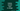# How to convert float to integer in Python## How to convert float to integer in Python:

In this post, we will learn how to convert a float to integer in python. We have different ways to do this conversion in Python. In this post, I will show you these methods one by one.

## Method 1: By using int():

int() function can take one floating point value and return the integer part. It trims the value after the decimal point.

Note that you might lose data if you use this function.

For example:

``````given_values = [1, 2, 2.3, 2.6, 3, 3.0]

for i in given_values:
print(f'{i} => {int(i)}')``````

It will print:

``````1 => 1
2 => 2
2.3 => 2
2.6 => 2
3 => 3
3.0 => 3``````

As you can see, it is not rounding the values.

We can also use math.floor and math.ceil.

## Method 2: math.floor and math.ceil:

### math.floor:

math.floor method rounds a number down to its nearest integer. Let’s take a look at the below example:

``````import math

given_values = [1, 2, 2.3, 2.6, 3, 3.0, 3.9, 4.1]

for i in given_values:
print(f'{i} => {math.floor(i)}')``````

If you run this, it will print the below output:

``````1 => 1
2 => 2
2.3 => 2
2.6 => 2
3 => 3
3.0 => 3
3.9 => 3
4.1 => 4``````

As you can see, it is rounding the number down to the nearest integer.

### math.ceil:

math.ceil returns the oppsite of math.floor. It rounds the number up to the nearest integer.

If we use math.ceil with the above array, it will give the below result:

``````import math

given_values = [1, 2, 2.3, 2.6, 3, 3.0, 3.9, 4.1]

for i in given_values:
print(f'{i} => {math.ceil(i)}')``````

It will print:

``````1 => 1
2 => 2
2.3 => 3
2.6 => 3
3 => 3
3.0 => 3
3.9 => 4``````

As you can see here, it returns the integer value by rounding the number up to the nearest.